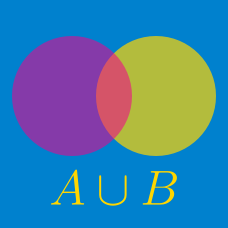Probability

# Sets - Complement

Consider the ground set $U= \{x \mid x \text{ is a positive integer less than } 33\}.$ If $A$ is a subset of $U$ and $A^c = \{x \mid x \text{ is a positive integer less than } 30\},$ what is the sum of all the elements in the set $A?$

Details and assumptions

You may choose to read the summary page on Set Notation.

Consider a set $U$ with $39$ elements. If $A$ is a subset of $U$ such that $\lvert A^c \rvert =9,$ how many elements are there in the set $A?$

Details and assumptions

You may choose to read the summary page on Set Notation.

Bella keeps a supply of red, blue, and black pens in a drawer. The drawer has 18 red pens, 18 blue pens, and 11 black pens. If Bella wants a red pen, what is the minimum number of pens she has to pull out from the drawer to make sure she gets a red pen?

How many of the integers from 1 to 100 inclusive are not perfect squares of an integer?

Consider the set $U=\{3,5,8,11,15,21\},$ and the subset $A=\{8,11\}$ of $U$. What is the sum of all the elements in the set $A^c?$

Details and assumptions

You may choose to read the summary page on Set Notation.

×